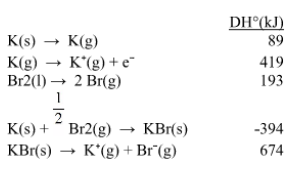# Problem: Use the data given below to construct a Born-Haber cycle to determine the electron affinity of Br.

🤓 Based on our data, we think this question is relevant for Professor Zimmer De Iuliis' class at TORONTO.

###### FREE Expert Solution

We’re being asked to calculate the electron affinity of bromine (Br), given the lattice energy of KBr.

Recall that lattice energy is the energy required to combine two gaseous ions into a solid ionic compound:

Mx+(g) + Ny–(g)  MyNx(s)

To calculate for lattice energy, we need to do the Born-Haber cycle for KBr. We start with the corresponding formation equation for KBr:

K(s) + ½ Br2(l)  KBr(s), ΔH˚f###### Problem Details

Use the data given below to construct a Born-Haber cycle to determine the electron affinity of Br.What scientific concept do you need to know in order to solve this problem?

Our tutors have indicated that to solve this problem you will need to apply the Born Haber Cycle concept. You can view video lessons to learn Born Haber Cycle. Or if you need more Born Haber Cycle practice, you can also practice Born Haber Cycle practice problems.

What is the difficulty of this problem?

Our tutors rated the difficulty ofUse the data given below to construct a Born-Haber cycle to ...as medium difficulty.

How long does this problem take to solve?

Our expert Chemistry tutor, Sabrina took 7 minutes and 32 seconds to solve this problem. You can follow their steps in the video explanation above.

What professor is this problem relevant for?

Based on our data, we think this problem is relevant for Professor Zimmer De Iuliis' class at TORONTO.